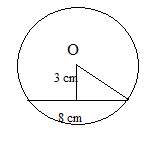# SSC CGL 2018 Practice Test Papers | Quantitative Aptitude (Day-39)

Dear Aspirants, Here we have given the Important SSC CGL Exam 2018 Practice Test Papers. Candidates those who are preparing for SSC CGL 2018 can practice these questions to get more confidence to Crack SSC CGL 2018 Examination.

[WpProQuiz 3330]

Click “Start Quiz” to attend these Questions and view Explanation

1) A chord of length 8 cm is at a distance of 3 cm from the centre of the circle. What is the area of the circle?

a) 79 cm2

b) 78.6 cm2

c) 60.9 cm2

d) 55.1 cm2

2) Find the length of the arc whose central angle is 135 o and radius of the circle is 42 cm.

a) 100 cm

b) 99 cm

c) 110 cm

d) 150 cm

3) A can do 2/3 of a job in 24 days. B is twice as efficient A. In how many days B will do half of the job?

a) 8 days

b) 7 days

c) 9 days

d) 10 days

4) In a division sum, the divisor is 3 times the quotient and twice the remainder. If the divisor and dividend are x and y respectively, then

a) 4x-y=3

b) 4y-2x=x2

c) x(2x+3)=6y

d) None of the above

5) In order to increase sales, a trader offered 20% discount on a bicycle. Hence profit per bicycle decreased by Rs. 180. Find the ratio of selling price and marked price.

a) 30: 51

b) 31: 45

c) 53: 47

d) 42: 49

6) If θ is the angle of first quadrant such that cosec4 θ = 17 + cot4 θ then what is the value of sinθ?

a) 1/3

b) 1/4

c) 1/9

d) 1/16

7) Find the average of all multiples of 7 below 300?

a) 150

b) 147

c) 130

d) 125

8) The average monthly salary of a group of sixteen people is Rs. 500. If three people of monthly salary of Rs. 1000, Rs. 1200 and rs.1400 join them and one person of monthly salary of Rs.800 leaves them, what’s the new average salary of the group?

a) 900

b) 600

c) 500

d) 800

9) Which digits should come in place of * and \$ if the number 4675*2\$ is divisible by both 5 & 8?

a) 4, 0
b) 4, 5
c) 1, 0
d) 8, 0

10) Simplify: 362+ 4= ? + 272

a) 510

b) 583

c) 430

d) 667Radius of circle= √ (42+32) = 5 cm

Area of circle = 22/7×5×5 = 78.6 cm2

Central angle of Arc = 135o

Central Angle of a circle = 360o

Circumference of circle = 2×22/7×42 = 264 cm

Arc length = 135/360 (Circumference of circle)

= 3/8 (Circumference of circle)

= 3/8(264) = 99 cm

A can do the whole work in 24×3/2= 36 days

B can do the whole work in 36/2=18 days

B will do half of the job in 18/2 =9 days

Quotient = x/3

Remainder = x/2

By remainder theorem,

Dividend= Quotient x divisor + remainder

Y = x2/3+x/2

Y = x(x/3+1/2)

6y = x(2x+3)

20% = 180

100% = 900

Marked Price = 900

Selling Price = 900 – 180 = 620

Ratio= 31: 45

cosec4θ = 17 + cot4θ

Cosec4θ – cot4θ = 17

(Cosec2θ – cot2θ)(Cosec2θ+ cot2θ) = 17

Cosec2θ + cosec2θ – 1 = 17

2/sin2θ = 18

sin2θ =1/9

sinθ =1/3

Average of multiples of 7 below 300 = (7+287)/2 = 147

Total salary of 16 people = 16×500 = 8000

Salary after adding new people= 8000 + 1000 + 1200 + 1400 – 800

=10800

Average salary of the new group= 10800/18= 600

Divisibility rule of 5= Last digit should be 0 or 5

Divisibility rule of 8= Last three digits should be divisible by 3

So the Answer is (1, 0)

362+ 42=? + 272

1296 + 16 – 729 = 583